## Lesson 3.5

Lesson 3.5
Testing Hypothesis about two means: Independent and
Large Samples.

In this lesson you will learn about testing hypothesis about two population
means (µ1 and µ2) and constructing confidence intervals for the difference
(µ1- µ2) between two population means. In doing this we will make the
following assumptions:
1.
The samples are independent. This means that the sample collected from one population is not related to the sample collected from the other population. The two sample sizes are large, n1 > 30 and n2 > 30. This means that the critical value (cv) and the test statistic (ts) both are z-values. A good example of testing a hypothesis involving two populations is the case of testing a drug for side effects. Two groups of people are used in testing a drug. The first group is called the “treatment group” who are actually treated with the medicine and the other group is called the control group who actually do not use the drug. Here the treatment group which is the first sample is not related to the control group which is the second sample. 1 and n1 to represent the sample mean, sample standard deviation and the sample size respectively. Similarly for sample 2
We use µ1 for the mean of the first population and µ2 for the mean of the
second population. The claim is always regarding µ1 and µ2, and we use the
two samples to test the claim. µ1 > µ2, µ1 < µ2, µ1 = µ2, µ1 ≠ µ2 are typical
claims using two populations.
The z-cv is obtained using the invNorm menu in TI-83
The z-ts is given by:
( x x ) − (µ − µ )
We use 2-SampZtest menu in TI-83 to obtain z-ts. This menu can be
accessed by:
[STAT],TESTS,.
We will use s1 and s2, the standard deviations of the samples in place of
σ1 and σ2 in the calculator.
Example 1:
The Anderson Pharmaceutical Company wants to test prilosec, a new
medicine for acid reflex disease. In order to test the effect of prilosec on
systolic blood pressure, 50 people with acid reflex disease are given prilosec
and 100 others are not. The systolic blood pressure is measured for each
subject and the sample statistic is as follows:
The head of research at Anderson claims that prilosec does not affect blood
pressure. Use a 0.01 level of significance to test this claim.
Solution:
Prilosec has no effect on systolic blood pressure means that the mean blood
pressure of those in the treatment group (µ1) equal to the mean blood
pressure of those in the control group (µ2).
1.
This is a two-tailed test. (tail area = α/2 = 0.005) Using area to the left = 0.005 in the invNorm menu gives: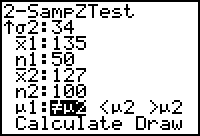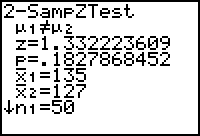z-cv = ±2.58
Access the 2-SampZTest by pressing [STAT],right arrow twice to
choose TESTS, . Now type in each required data, choose two-tailed
test and down arrow to Calculate and [ENTER]. The calculator
display is shown below. This gives the z-ts = 1.33
z-cv, z-ts and the critical regions are shown in fig. 2 below. The z-ts does not fall in the critical region, so we fail to reject the Ho: Claim is the same as the null hypothesis and we fail to reject the Ho. There is not enough evidence to reject the claim that prilosec does not affect systolic blood pressure.
Confidence Interval for the difference between the two means:
A confidence interval for µ1 -µ2 is of the form:
( x x ) − E < µ − µ < (x x ) + E where E is the margin or error and is given by: As the sample sizes are large, we replace σ1 and σ2 by s1 and s2 respectively.
Example 2:
Using the samples in example 1, construct the 99% confidence interval for µ1 - µ2.
Solution:
1.
α = 0.01, therefore, α/2 = 0.005 and zα/2 = 2.58 ( x x ) − E < µ − µ < (x x ) + E 8.0 −15.5 < µ − µ < 8.0 + 15.5 Since this interval contains zero, there is not enough evidence to reject the claim that µ1 – µ2 = 0
Example 3:
A study was conducted to compare the weights of college freshmen who commute from home and those who are resident on college campus. For 750 freshmen who commute from home, the mean is 72.5 kg and the standard deviation is 14.8 kg. The mean weight of 1250 resident freshmen is 68 kg with a standard deviation of 12.7 kg. (a) Use a 0.01 level of significance to test the claim that the resident freshmen come from a population with a mean that is less than the mean for the commuting freshmen. (b) Construct the 99% confidence interval for the difference between the means of the two population of students.
Solution:
(a):
The sample data can be summarized as follows: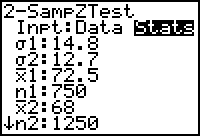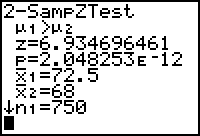The statement of claim is always made starting with population 1 1. This is a right tail test. (tail area = α = 0.01) Using area to the left = 1 – 0.01 in the invNorm menu, we get:
z-cv = 2.33
Using the sample data in the 2-SampZTest menu, we get:
z-ts = 6.93
[STAT],TESTS,
The values of z-cv, z-ts and critical region are shown in the figure below. Fig. 4. z-ts is inside the critical region The z-ts is inside the critical region, and so we reject the null hypothesis. The claim is the same as the alternative hypothesis, and we reject the null hypothesis. Therefore, we support the alternative hypothesis and hence the claim. There is enough evidence to support the claim that the resident
freshmen come from a population with a mean that is less than
the mean for the commuting freshmen.

99% confidence gives α = 0.01, and α/2 = 0.005. Therefore zα/2 = 2.58 (x x ) − E < µ − µ < (x x ) + E This interval supports the claim that µ1 > µ2.
Example 4:
A recent report in Charlotte Sun Herald claimed that Charlotte county students performed better in the math section of the 10th grade FCAT than students from Lee County. A sample of 35 10th graders from Charlotte has the following math scores. 79, 85, 92, 55, 62, 78, 89, 88, 59, 92, 85, 87, 91, 62, 69, 71, 51, 42, 69, 74, 53, 84, 91, 55, 69, 72, 82, 95, 60, 54, 42, 55, 65, 71, 80 A sample of 40 10th graders from Lee County has the following math scores. 88, 51, 42, 91, 75, 68, 44, 75, 78, 64, 62, 69, 92, 44, 38, 72, 69, 84, 89, 53, 49, 93, 99, 42, 53, 63, 74, 82, 51, 41, 32, 67, 75, 83, 79, 50, 44, 69, 70, 58 At the 0.04 significant level test the claim of the Sun Herald. Construct the 96% confidence interval for the difference between the two mean scores.
Solution: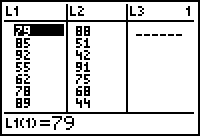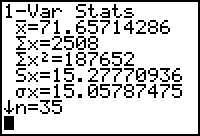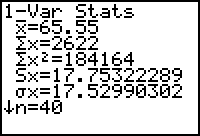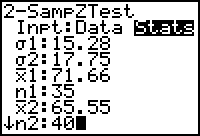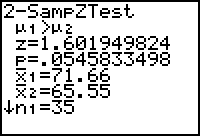Clear existing lists L1 and L2.
Use the data to construct lists L1 and L2 and do 1-Var Stats on these to
obtain the sample data.
[STAT],[ENTER]
[STAT],CALC,[ENTER],[2nd]
[STAT],CALC,[ENTER],[2nd]
The data can be summarized as follows:: This is a right tailed test. (area to the left = 1 – 0.04) Using the sample data in the 2-SampZTest, we get z-ts = 1.6
The values of z-cv, z-ts and the critical region are shown in the figure below.Fig. 6. z-ts is not in the critical region. The z-ts is not inside the critical region, so we fail to reject the null hypothesis. The claim = H1: and we fail to reject the Ho:, therefore we fail to support H1 and hence the claim. There is not enough evidence to support the claim that Charlotte 10th graders performed better than Lee 10th graders. (x x ) − E < µ − µ < (x x ) + E 6.11 − 7.82 < µ − µ < 6.11 + 7.82 Inferences about Two Proportions.
In this section we will test hypothesis made about two population proportions and construct confidence interval about the difference between the two population proportions. Here we will assume that the two samples are randomly selected and are independent. The following are the notations we will use in this section. x1 = number of success in the sample x2 = the number of successes in the
Typical claims involving proportions are p1 > p2, p1 < p2, p1 = p2 and so on.
The critical value in tests involving proportion is the z-cv and it is obtained
using the invNorm menu in TI-83.
The test statistic is the z-ts which is defined as follows ;
( ˆp − ˆp ) − ( p p )
We will use the 2-PropZTest to obtain z-ts in this case. This menu can be
accessed by pressing [STAT],right arrow twice to select TESTS,. Type in
the required data, choose the type of test and down arrow to choose calculate
and [ENTER].
Confidence Intervals:
The confidence interval for the difference between population proportions p1
– p2 is constructed as follows:
( ˆp − ˆp ) − E < ( p p ) < ( ˆp − ˆp ) + E where E is given by:
Example 5:
A U.S. Department of Education report included the claim that “girls are less likely to be dropped out of school than boys before they are 18.” Sample data consisted of 1350 girls, 238 of them dropped out of school before they completed 18 and 2358 boys, 422 of them dropped out of school before they were 18. Use a 0.05 significance level to test this claim. Construct the 95% confidence interval for the difference between the two population proportions.
Solution:
Here population 1 consists of girls who dropped out of school before 18, and
population 2 consists of boys who dropped out of school before 18. The
claim is that the proportion of girls dropping out of school before 18 is less
than the proportion of boys dropping out of school before 18. The sample
data is as follows:
This is a left tail test. (Since no significance level is specified, we choose 0.05 and this is the tail area.) Using area to the left = 0.05 in the invNorm menu, we get:
z-cv = -1.645
Use the sample data in the 2-PropZTest and obtain z-ts as shown in
the figure below. z-ts = - 0.204
[STAT],TESTS,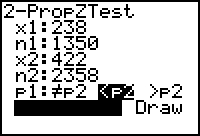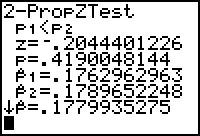The values of z-cv, z-ts and the critical region are shown in the figure below. z-ts = - 0.204
cv = - 1.645
Fig. 8. z-ts is outside the critical region. The z-ts is outside the critical region, and so we fail to reject the null hypothesis. Claim = H1 and we fail to reject Ho, and so we fail to support H1 and
hence the claim.
There is not enough evidence to support the claim that proportion
of girls dropping out of school is less than the proportion of boys
dropping out of school.

95% confidence level means that α = 0.05 and α/2 = 0.025. Using area to the left as 0.025 in invNorm menu, we get zα/2 = 1.96
ˆp − ˆp = 0.176 − 0.179 = −0.003 − E < ( p1 − p ) = - 0.003 – 0.026 < (p1 – p2) < - 0.003 + 0.026 This result supports the conclusion of the hypothesis test as p1 – p2 is likely
to contain zero.

Example 6
:
A public relations expert and consultant for the television industry is planning a strategy to influence voter perception of government regulation of television programs. In a survey it is found that 35% of 552 Democrats believe that the government should regulate television programs, compared to 41% of the 417 Republicans surveyed. (a) At the 0.05 significance level, test the claim that there is no difference between the proportions of Democrats and Republicans who believe in government regulation of television programs. (b) Construct a 95% confidence interval for the difference between the proportions of Democrats and Republicans who believe that government
should regulate television programs.
Solution:

There is no difference between the two proportions means that p1 = p2.
Summary of data: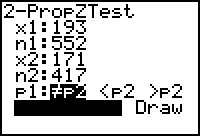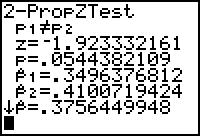This is a two-tailed test. Tail area = so α/2 = 0.025 Using area to the left = 0.025 in invNorm, gives z-cv = ±1.96
Using the sample data in the 2-PropZTest menu, we get z-ts = -1.92
as shown in fig. 9 below.
[STAT],TESTS,
The z-cv, z-ts and the critical region are shown in fig. 10 below. Fig. 10. z-ts is not inside the critical region. z-ts is not inside the critical region, so we fail to reject the null hypothesis. Claim = Ho and we fail to reject Ho, so we fail to reject the claim.
There is not enough evidence to reject the claim that the two
proportions are the same.

95% confidence means that α = 0.05 and α/2 = 0.025 Using area to the left = 0.025 in invNorm menu, we get zα/2 = 1.96
( ˆp − ˆp ) − E < ( p p ) < ( ˆp − ˆp ) + E = (0.35 – 0.41) - 0.062 < p1 – p2) < (0.35 – 0.41) + 0.062 = -0.122 < (p1 – p2) < 0.002
Since the confidence interval contains zero, p1 – p2 = 0 is a strong
possibility.
Example 7:
A random sample of 216 registered voters in Florida showed that 125 of
them voted in the 2000 presidential elections. A random sample of 288
registered voters in Texas showed that 141 of them voted in the presidential
elections of 2000.
(a)
At a 5% significance level, test the claim that the proportion of registered voters who voted in Florida is greater than the proportion of registered voters who voted in Texas. Construct the 95% confidence interval for the difference between the voter turnovers in Florida and in Texas.
Solution:
The data can be summarized as follows: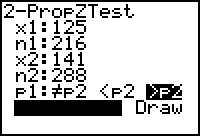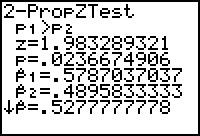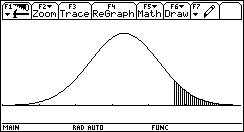This is a right-tail test. Tail area = α = 0.05 Using area to the left = 1 – 0.05, z-cv = 1.64
Using the sample data in the 2-PropZTest menu, z-ts = 1.983
z-ts = 1.98
z-cv = 1.64
z-ts is inside the critical region. Therefore, we reject the null hypothesis. Claim = H1, and we reject the null hypothesis. Therefore, we support the alternate hypothesis and hence the claim. There is enough evidence to support the claim that the proportion of voter turnout in Florida is greater than the proportion of voter turnout in Texas. Degree of confidence is 95% means that α = 0.05 and α/2 = 0.025 ( ˆp − ˆp ) − E < p p < ( ˆp − ˆp ) + E (0.579 − 0.490) − 0.088 < p p < (0.579 − 0.490) + 0.088 0.011 < p p < 0.177 Homework:
PROJECT:

You are required to undertake a comparative study about the proportion of high school graduates who go to college from charlotte High and Port charlotte High. (You can pick two other schools of your choice). (i) Collect appropriate data. (The school guidance counselor will be able to provide you with the data that you need). (iii) Organize and summarize your data. (iv) Do a 10-step hypothesis test. (v) Make a clear statement about your conclusion.

Source: http://gmanacheril.com/STA2023/Text%20Materials/Lesson%203.5.pdf

### exiqon.com

In situ hybridisation on yeast with Locked Nucleic Acid in situ detection probesProtocol prepared by Dr. Torben Heick, Department of Molecular Biology Aarhus University, DenmarkThe protocol originates from the Singer laboratory (Long et al. (1997) Science 277, 383-387) and has been modified for yeast, Saccharomyces cerevisiae, cell cultures subjected to a heat-shock treatment. However, the outli

### Pe7734038-1 naproxen sodium 275-550mg.pmd

PE7734038-1 Naproxen Sodium Tablets 275-550mg Cut Size : 270 x 420 mm 6 pt helv condensed Pharma code: 640 Date: 11.03.2008 (Front) Naproxen Naproxen Sodium Tablets USP • For the relief of the signs and symptoms of rheumatoid arthritis Medication Guide • For the relief of the signs and symptoms of osteoarthritis • For the relief of the signs and symptoms of ankylosing spondyli